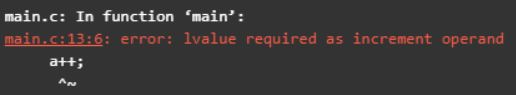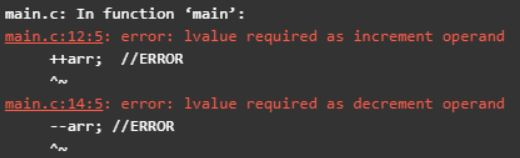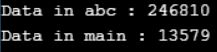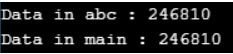# Array Exercise in C

## Array Exercise in C

In this article, I am going to discuss Array Exercise in C with Examples. Please read our previous articles, where we discussed Functions using Array in C Program.

##### What will be the output of the below program?
```#include<stdio.h>
int main()
{
char x[]="CTutorials", y[]="CTutorials";
if(x==y)
{
printf("Strings are Equal");
}
else
{
printf("Strings are not Equal");
}
}```

Output: Strings are not Equal

This is because in the above program we are comparing the base address of ‘x’ and ‘y’ and they are not the same. Therefore, the program shows not Equal output.

##### What will be the output of the below program?
```#include<stdio.h>
int main(){
char x[]="Hi\0Hello";
printf("%d %d", strlen(x), sizeof(x));
return 0;
}```

Output: 2 9

The strlen(x) function is used for finding the length of string ‘x’. In the program, the length of the string is the count of the character up to ‘\0’. Hence the string length output is 2.

The sizeof(x) function is used for finding the size of string ‘x’. In program sizeof() returns the size of the complete array. Hence the size of the array output is 9. Therefore, the combined output of the program is 2 9.

##### What will be the output of the below program?
```#include<stdio.h>
int main(){
int x[] = {100,200,300};
printf("%d", *x +1);
return 0;
}```

Output: 101

In the program *x refers to 100 and adding a 1 to *x gives 101. Therefore, the output is 101.

##### What will be the output of the below program?
```#include<stdio.h>
int main(){
char a[] = "C++";
printf("%s ",a);
a++;
printf("%s",a);
return 0;
}```
###### Output:Compile error: In the program a refers to constant address and the constant address variable is not allowed to be incremented. Therefore, the program will generate a compile error in the output.

##### What will be the output of the below program?
```#include<stdio.h>
int main(){
int arr={20};
printf("%d\n", 0[arr]);
return 0;
}```

Output: 20

Step1: int arr={20}; The variable arr is declared as an integer array with size of ‘3’ and it’s first element is initialized with value ’20′(means arr=20)

Step2: printf(“%d\n”, 0[arr]); It prints the first element value of variable ‘arr’. Therefore, the output of the program is 20.

##### What will be the output?
```#include<stdio.h>
int main(){
int arr = {1, 11, 21, 31, 41};
int *ptr = (int*) NULL;
ptr = &arr;
++ptr;
--*ptr;
--ptr;
++*ptr;
printf("%d %d", arr, arr);
return 0;
}```

Output: 2 10

When we are working with * and pre-operator. Here both contain equal priority. When equal priority occurs, if it is a binary operator then evaluates towards from left to right and if it is a unary operator then evaluates towards from right to left.

##### What will be the output?
```#include<stdio.h>
int main(){
int arr = {3, 13, 23, 33, 43};
int *ptr = NULL;
ptr = arr; //ptr = &arr
++ptr;
--*ptr;
--ptr;
++*ptr;
printf("%d %d", arr, arr);
return 0;
}```

Output: 4 12

An array is an implicit pointer variable that always holds the base address of an array. Array name always gives the base address of an array i.e. &arr. arr+1 gives next address of an array i.e. &arr

##### What will be the output?
```#include<stdio.h>
int main(){
int arr[] = {4, 14, 24, 34, 44};
int *ptr = arr + 1; // ptr = &arr;
++ptr;
--*ptr;
--ptr;
++*ptr;
printf("%d %d %d", ptr, ptr, ptr[-1]);
return 0;
}```

Output: 15 23 4

On pointer variable when we are applying subscript operator then index value will be mapped with current pointer data and applies ‘*’ operator.

##### What will be the output?
```#include<stdio.h>
int main(){
int arr[] = {5, 15, 25, 35, 45};
int *ptr = NULL;
ptr = &arr;
++ptr;
++*ptr;
printf("%d %d %d %d", arr, *(arr+2), 2[arr], *(2 + arr));
return 0;
}```

Output: 26 26 26 26

When we are working with an array, all element information we are not holding programmatically. An array is an implicit pointer variable that always holds the base address of an array i.e. single-cell information only (first element address).

According to the architecture of the array always index value will be mapped with the base address of an array. When we are applying the subscript operator on the array, the index value will be mapped with the base address of an array and applied indirection (*) operator, so by using that address correspondent data will be accessible.

Note: Array index always should start with ‘0’ only because if we are starting with 1 then in place of accessing the first element it will access the second element.

##### What will be the output?
```#include<stdio.h>
int main(){
int arr[] = {6, 16, 26, 36, 46};
++arr;  //ERROR
++*arr;
--arr; //ERROR
--*arr;
printf("%d %d", arr, arr);
return 0;
}
```
###### Output:An array is an implicit constant pointer variable so incrementation and decrementation on the array are not allowed.

##### What will be the output?
```#include<stdio.h>
void abc(int a)
{
++a;
printf("%d", a);
}
int main()
{
int i, arr = {1,3,5,7,9};
printf("Data in abc : ");
for(i = 0; i<5; i++)
abc(arr[i]);
printf("\nData in main : ");
for(i = 0; i<5; i++)
printf("%d", arr[i]);
return 0;
}```
###### Output:In the above program array elements are passing by using call by value mechanism that’s why no modification of abc() function will pass back to the main() function. In implementation when we require to pass modification back to the main function then go for the call by address mechanism. When we are implementing call by address mechanism then we are required to pass an address of an element.

##### What will be the output?
```#include<stdio.h>
void abc(int* ptr)
{
int a;
a= *ptr;
*ptr = ++a;
printf("%d", *ptr);
}
int main()
{
int i, arr = {1,3,5,7,9};
printf("Data in abc : ");
for(i = 0; i<5; i++)
abc(&arr[i]);
printf("\nData in main : ");
for(i = 0; i<5; i++)
printf("%d", arr[i]);
return 0;
}```
###### Output:In the above program elements are passing by using call by address mechanism that’s why all modifications of abc() function will be carried back to the main() function. In any programming language, it is not possible to pass the entire array as an argument to the function because it creates lots of memory wastage.

In implementation when we are required to pass the entire array as an argument then we need to pass the base address of the array along with the size. If we know the base address by using index value with the help of size complete array data we can access it from outside the function.